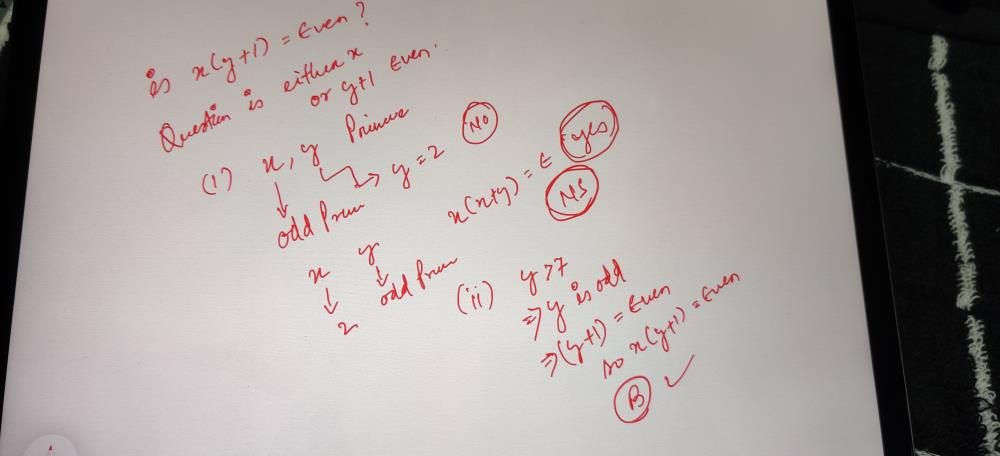Courses

# If x and y are integers, is x (y + 1) an even numb... moreer?1)xand y are prime numbers.2)y > 7 ?a)Exactly one of the statements can answer the questionb)Both statements are required to answer the questionc)Each statement can answer the question individuallyd)More information is required as the information provided is insufficient to answer the questionCorrect answer is option 'B'. Can you explain this answer? Related Test: Test: Even And Odds-1

## GMAT QuestionPreitee Ranjan Pradhan Jun 06, 2020This discussion on If x and y are integers, is x (y + 1) an even numb... moreer?1)xand y are prime numbers.2)y > 7 ?a)Exactly one of the statements can answer the questionb)Both statements are required to answer the questionc)Each statement can answer the question individuallyd)More information is required as the information provided is insufficient to answer the questionCorrect answer is option 'B'. Can you explain this answer? is done on EduRev Study Group by GMAT Students. The Questions and Answers of If x and y are integers, is x (y + 1) an even numb... moreer?1)xand y are prime numbers.2)y > 7 ?a)Exactly one of the statements can answer the questionb)Both statements are required to answer the questionc)Each statement can answer the question individuallyd)More information is required as the information provided is insufficient to answer the questionCorrect answer is option 'B'. Can you explain this answer? are solved by group of students and teacher of GMAT, which is also the largest student community of GMAT. If the answer is not available please wait for a while and a community member will probably answer this soon. You can study other questions, MCQs, videos and tests for GMAT on EduRev and even discuss your questions like If x and y are integers, is x (y + 1) an even numb... moreer?1)xand y are prime numbers.2)y > 7 ?a)Exactly one of the statements can answer the questionb)Both statements are required to answer the questionc)Each statement can answer the question individuallyd)More information is required as the information provided is insufficient to answer the questionCorrect answer is option 'B'. Can you explain this answer? over here on EduRev! Apart from being the largest GMAT community, EduRev has the largest solved Question bank for GMAT.